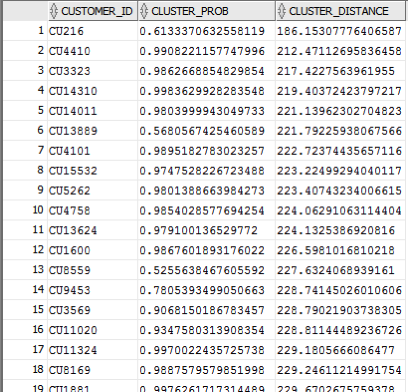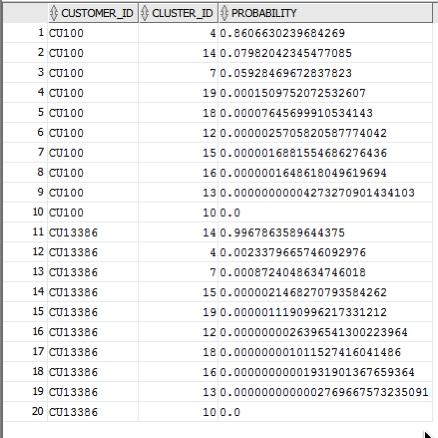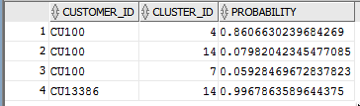# data mining

### Demographics vs Psychographics for Machine Learning

Posted on Updated on

When preparing data for data science, data mining or machine learning projects you will create a data set that describes the various characteristics of the subject or case record. Each attribute will contain some descriptive information about the subject and is related to the target variable in some way.

In addition to these attributes, the data set will be enriched with various other internal/external data to complete the data set.

Some of the attributes in the data set can be grouped under the heading of Demographics. Demographic data contains attributes that explain or describe the person or event each case record is focused on. For example, if the subject of the case record is based on Customer data, this is the “Who” the demographic data (and features/attributes) will be about. Examples of demographic data include:

• Age range
• Marital status
• Number of children
• Household income
• Occupation
• Educational level

These features/attributes are typically readily available within your data sources and if they aren’t then these name be available from a purchased data set.

Additional feature engineering methods are used to generate new features/attributes that express meaning is different ways. This can be done by combining features in different ways, binning, dimensionality reduction, discretization, various data transformations, etc. The list can go on.

The aim of all of this is to enrich the data set to include more descriptive data about the subject. This enriched data set will then be used by the machine learning algorithms to find the hidden patterns in the data. The richer and descriptive the data set is the greater the likelihood of the algorithms in detecting the various relationships between the features and their values. These relationships will then be included in the created/generated model.

Another approach to consider when creating and enriching your data set is move beyond the descriptive features typically associated with Demographic data, to include Pyschographic data.

Psychographic data is a variation on demographic data where the feature are about describing the habits of the subject or customer.  Demographics focus on the “who” while psycographics focus on the “why”. For example, a common problem with data sets is that they describe subjects/people who have things in common. In such scenarios we want to understand them at a deeper level. Psycographics allows us to do this. Examples of Psycographics include:

• Lifestyle activities
• Evening activities
• Purchasing interests – quality over economy,  how environmentally concerned are you
• How happy are you with work, family, etc
• Social activities and changes in these
• What attitudes you have for certain topic areas
• What are your principles and beliefs

The above gives a far deeper insight into the subject/person and helps to differentiate each subject/person from each other, when there is a high similarity between all subjects in the data set. For example, demographic information might tell you something about a person’s age, but psychographic information will tell you that the person is just starting a family and is in the market for baby products.

### Managing imbalanced Data Sets with SMOTE in Python

Posted on Updated on

When working with data sets for machine learning, lots of these data sets and examples we see have approximately the same number of case records for each of the possible predicted values. In this kind of scenario we are trying to perform some kind of classification, where the machine learning model looks to build a model based on the input data set against a target variable. It is this target variable that contains the value to be predicted. In most cases this target variable (or feature) will contain binary values or equivalent in categorical form such as Yes and No, or A and B, etc or may contain a small number of other possible values (e.g. A, B, C, D).

For the classification algorithm to perform optimally and be able to predict the possible value for a new case record, it will need to see enough case records for each of the possible values. What this means, it would be good to have approximately the same number of records for each value (there are many ways to overcome this and these are outside the score of this post). But most data sets, and those that you will encounter in real life work scenarios, are never balanced, as in having a 50-50 split. What we typically encounter might be a 90-10, 98-2, etc type of split. These data sets are said to be imbalanced.The image above gives examples of two approaches for creating a balanced data set. The first is under-sampling. This involves reducing the class that contains the majority of the case records and reducing it to match the number of case records in the minor class. The problems with this include, the resulting data set is too small to be meaningful, the case records removed could contain important records and scenarios that the model will need to know about.

The second example is creating a balanced data set by increasing the number of records in the minority class. There are a few approaches to creating this. The first approach is to create duplicate records, from the minor class, until such time as the number of case records are approximately the same for each class. This is the simplest approach. The second approach is to create synthetic records that are statistically equivalent of the original data set. A commonly technique used for this is called SMOTE, Synthetic Minority Oversampling Technique. SMOTE uses a nearest neighbors algorithm to generate new and synthetic data we can use for training our model. But one of the issues with SMOTE is that it will not create sample records outside the bounds of the original data set. As you can image this would be very difficult to do.

The following examples will illustrate how to perform Under-Sampling and Over-Sampling (duplication and using SMOTE) in Python using functions from Pandas, Imbalanced-Learn and Sci-Kit Learn libraries.

NOTE: The Imbalanced-Learn library (e.g. SMOTE)requires the data to be in numeric format, as it statistical calculations are performed on these. The python function get_dummies was used as a quick and simple to generate the numeric values. Although this is perhaps not the best method to use in a real project. With the other sampling functions can process data sets with a sting and numeric.

Data Set:  Is the Portuaguese Banking data set and is available on the UCI Data Set Repository, and many other sites. Here are some basics with that data set.

```import warnings

import pandas as pd
import numpy as np
import matplotlib.pyplot as plt
get_ipython().magic('matplotlib inline')

# import dataset

# get basic details of df (num records, num features)
df.shape```
`df['y'].value_counts() # dataset is imbalanced with majority of class label as "no".`
```no     36548
yes     4640
Name: y, dtype: int64```
```#print bar chart
df.y.value_counts().plot(kind='bar', title='Count (target)');```Example 1a – Down/Under sampling the majority class y=1 (using random sampling)

```count_class_0, count_class_1 = df.y.value_counts()

# Divide by class
df_class_0 = df[df['y'] == 0] #majority class
df_class_1 = df[df['y'] == 1] #minority class

# Sample Majority class (y=0, to have same number of records as minority calls (y=1)
df_class_0_under = df_class_0.sample(count_class_1)
# join the dataframes containing y=1 and y=0
df_test_under = pd.concat([df_class_0_under, df_class_1])

print('Random under-sampling:')
print(df_test_under.y.value_counts())
print("Num records = ", df_test_under.shape)

df_test_under.y.value_counts().plot(kind='bar', title='Count (target)');```
```Random under-sampling:
1    4640
0    4640
Name: y, dtype: int64
Num records =  9280
```Example 1b – Down/Under sampling the majority class y=1 using imblearn

```from imblearn.under_sampling import RandomUnderSampler

X = df_new.drop('y', axis=1)
Y = df_new['y']

rus = RandomUnderSampler(random_state=42, replacement=True)
X_rus, Y_rus = rus.fit_resample(X, Y)

df_rus = pd.concat([pd.DataFrame(X_rus), pd.DataFrame(Y_rus, columns=['y'])], axis=1)

print('imblearn over-sampling:')
print(df_rus.y.value_counts())
print("Num records = ", df_rus.shape)

df_rus.y.value_counts().plot(kind='bar', title='Count (target)');```

[same results as Example 1a]

Example 1c – Down/Under sampling the majority class y=1 using Sci-Kit Learn

```from sklearn.utils import resample

print("Original Data distribution")
print(df['y'].value_counts())

# Down Sample Majority class
down_sample = resample(df[df['y']==0],
replace = True, # sample with replacement
n_samples = df[df['y']==1].shape, # to match minority class
random_state=42) # reproducible results

# Combine majority class with upsampled minority class
train_downsample = pd.concat([df[df['y']==1], down_sample])

# Display new class counts
print('Sci-Kit Learn : resample : Down Sampled data set')
print(train_downsample['y'].value_counts())
print("Num records = ", train_downsample.shape)
train_downsample.y.value_counts().plot(kind='bar', title='Count (target)');
```

[same results as Example 1a]

Example 2 a – Over sampling the minority call y=0 (using random sampling)

```df_class_1_over = df_class_1.sample(count_class_0, replace=True)
df_test_over = pd.concat([df_class_0, df_class_1_over], axis=0)

print('Random over-sampling:')
print(df_test_over.y.value_counts())

df_test_over.y.value_counts().plot(kind='bar', title='Count (target)');```
```Random over-sampling:
1    36548
0    36548
Name: y, dtype: int64```Example 2b – Over sampling the minority call y=0 using SMOTE

```from imblearn.over_sampling import SMOTE

print(df_new.y.value_counts())
X = df_new.drop('y', axis=1)
Y = df_new['y']

sm = SMOTE(random_state=42)
X_res, Y_res = sm.fit_resample(X, Y)

df_smote_over = pd.concat([pd.DataFrame(X_res), pd.DataFrame(Y_res, columns=['y'])], axis=1)

print('SMOTE over-sampling:')
print(df_smote_over.y.value_counts())

df_smote_over.y.value_counts().plot(kind='bar', title='Count (target)');```

[same results as Example 2a]

Example 2c – Over sampling the minority call y=0 using Sci-Kit Learn

```from sklearn.utils import resample

print("Original Data distribution")
print(df['y'].value_counts())

# Upsample minority class
train_positive_upsample = resample(df[df['y']==1],
replace = True, # sample with replacement
n_samples = train_zero.shape, # to match majority class
random_state=42) # reproducible results

# Combine majority class with upsampled minority class
train_upsample = pd.concat([train_negative, train_positive_upsample])

# Display new class counts
print('Sci-Kit Learn : resample : Up Sampled data set')
print(train_upsample['y'].value_counts())
train_upsample.y.value_counts().plot(kind='bar', title='Count (target)');```

[same results as Example 2a]

### Cluster Distance using SQL with Oracle Data Mining – Part 4

Posted on Updated on

This is the fourth and last blog post in a series that looks at how you can examine the details of predicted clusters using Oracle Data Mining. In the previous blog posts I looked at how to use CLUSER_ID, CLUSTER_PROBABILITY and CLUSTER_SET.

In this blog post we will look at CLUSTER_DISTANCE. We can use the function to determine how close a record is to the centroid of the cluster. Perhaps we can use this to determine what customers etc we might want to focus on most. The customers who are closest to the centroid are one we want to focus on first. So we can use it as a way to prioritise our workflows, particularly when it is used in combination with the value for CLUSTER_PROBABILITY.

Here is an example of using CLUSTER_DISTANCE to list all the records that belong to Cluster 14 and the results are ordered based on closeness to the centroid of this cluster.

```SELECT customer_id,
cluster_probability(clus_km_1_37 USING *) as cluster_Prob,
cluster_distance(clus_km_1_37 USING *) as cluster_Distance
FROM   insur_cust_ltv_sample
WHERE   cluster_id(clus_km_1_37 USING *) = 14
order by cluster_Distance asc;
```

Here is a subset of the results from this query.When you examine the results you may notice that the records that is listed first and closest record to the centre of cluster 14 has a very low probability. You need to remember that we are working in a N-dimensional space here. Although this first record is closest to the centre of cluster 14 it has a really low probability and if we examine this record in more detail we will find that it is at an overlapping point between a number of clusters.

This is why we need to use the CLUSTER_DISTANCE and CLUSTER_PROBABILITY functions together in our workflows and applications to determine how we need to process records like these.

### Cluster Sets using SQL with Oracle Data Mining – Part 3

Posted on Updated on

This is the third blog post on my series on examining the Clusters that were predicted by an Oracle Data Mining model. Check out the previous blog posts.

In the previous posts we were able to list the predicted cluster for each record in our data set. This is the cluster that the records belonged to the most. I also mentioned that a record could belong to many clusters.

So how can you list all the clusters that the a record belongs to?

You can use the CLUSTER_SET SQL function. This will list the Cluster Id and a probability measure for each cluster. This function returns a array consisting of the set of all clusters that the record belongs to.

The following example illustrates how to use the CLUSTER_SET function for a particular cluster model.

```SELECT t.customer_id, s.cluster_id, s.probability
FROM   (select customer_id, cluster_set(clus_km_1_37 USING *) as Cluster_Set
from   insur_cust_ltv_sample
WHERE  customer_id in ('CU13386', 'CU100')) T,
TABLE(T.cluster_set) S
order by t.customer_id, s.probability desc;
```

The output from this query will be an ordered data set based on the customer id and then the clusters listed in descending order of probability. The cluster with the highest probability is what would be returned by the CLUSTER_ID function. The output from the above query is shown below.If you would like to see the details of each of the clusters and to examine the differences between these clusters then you will need to use the CLUSTER_DETAILS function (see previous blog post).

You can specify topN and cutoff to limit the number of clusters returned by the function. By default, both topN and cutoff are null and all clusters are returned.

– topN is the N most probable clusters. If multiple clusters share the Nth probability, then the function chooses one of them.

– cutoff is a probability threshold. Only clusters with probability greater than or equal to cutoff are returned. To filter by cutoff only, specify NULL for topN.

You may want to use these individually or combined together if you have a large number of customers. To return up to the N most probable clusters that are greater than or equal to cutoff, specify both topN and cutoff.

The following example illustrates using the topN value to return the top 4 clusters.

```SELECT t.customer_id, s.cluster_id, s.probability
FROM   (select customer_id, cluster_set(clus_km_1_37, 4, null USING *) as Cluster_Set
from   insur_cust_ltv_sample
WHERE  customer_id in ('CU13386', 'CU100')) T,
TABLE(T.cluster_set) S
order by t.customer_id, s.probability desc;
```

and the output from this query shows only 4 clusters displayed for each record.Alternatively you can select the clusters based on a cut off value for the probability. In the following example this is set to 0.05.

```SELECT t.customer_id, s.cluster_id, s.probability
FROM   (select customer_id, cluster_set(clus_km_1_37, NULL, 0.05 USING *) as Cluster_Set
from   insur_cust_ltv_sample
WHERE  customer_id in ('CU13386', 'CU100')) T,
TABLE(T.cluster_set) S
order by t.customer_id, s.probability desc;
```

and the output this time looks a bit different.Finally, yes you can combine these two parameters to work together.

SELECT t.customer_id, s.cluster_id, s.probability
FROM (select customer_id, cluster_set(clus_km_1_37, 2, 0.05 USING *) as Cluster_Set
from insur_cust_ltv_sample
WHERE customer_id in (‘CU13386’, ‘CU100’)) T,
TABLE(T.cluster_set) S
order by t.customer_id, s.probability desc;